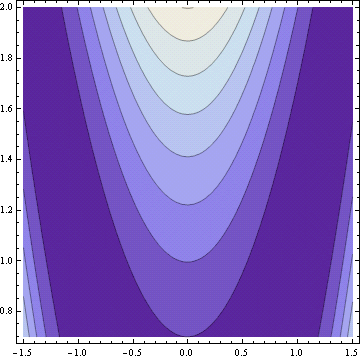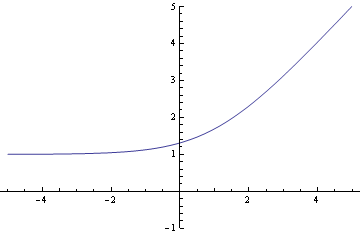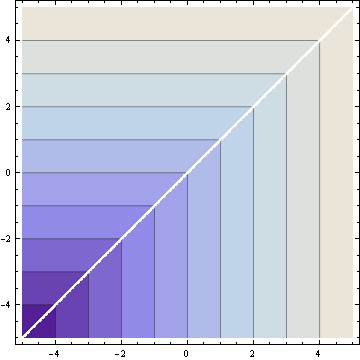# Designed from the inside out

The most recent episode of the Plus Maths podcast describes how the London Velodrome was designed. Being a math podcast, it focuses on the optimization problems involved in the design and the finite element modeling of the structure.

The beautiful shape of the building was not an initial goal but rather a consequence of design decisions that began with the track and worked outward.

It’s perhaps surprising, given the pragmatic design concerns of optimizing the experience of people using the velodrome, maximizing the efficiency of the building, all within the constraints of the construction methods, the design process has led to a stunningly beautiful roof that almost echos the shape of the track.

… it’s a happy by-product of the design. There was nothing intentional in the design [of the roof] that we wanted it to look like the track. … it’s the opposite if the track …Image by Richard Davies via the Plus Math article How the velodrome found its form.

Related post: Mathematics behind the Olympic water cube

# Markov chains don’t converge

I often hear people often say they’re using a burn-in period in MCMC to run a Markov chain until it converges. But Markov chains don’t converge, at least not the Markov chains that are useful in MCMC. These Markov chains wander around forever exploring the domain they’re sampling from. Any point that makes a “bad” starting point for MCMC is a point you might reach by burn-in.

Not only that, Markov chains can’t remember how they got where they are. That’s their defining property. So if your burn-in period ends at a point x, the chain will perform exactly as if you had simply started at x.

When someone says a Markov chain has converged, they may mean that the chain has entered a high-probability region. I’ll explain in a moment why that’s desirable. But the belief/hope is that a burn-in period will put a Markov chain in a high-probability region. And it probably will, but there are a couple reasons why this isn’t necessarily the best thing to do.

1. Burn-in may be inefficient. Casting aside worries that burn-in may not do what you want, it can be an inefficient way to find a high-probability region. MCMC isn’t designed to optimize a density function but rather to sample from it.

Why use burn-in? MCMC practitioners often don’t know how to do optimization, and in any case the corresponding optimization problem may be difficult. Also, if you’ve got the MCMC code in hand, it’s convenient to use it to find a starting point as well as for sampling.

So why does it matter whether you start your Markov chain in a high-probability region? In the limit, it doesn’t matter. But since you’re averaging some function of some finite number of samples, your average will be a better approximation if you start at a typical point in the density you’re sampling. If you start at a low probability location, your average may be more biased.

Samples from Markov chains don’t converge, but averages of functions applied to these samples may converge. When someone says a Markov chain has converged, they may mean they’re at a point where the average of a finite number of function applications will be a better approximation of the thing they want to compute than if they’d started at a low probability point.

It’s not just a matter of imprecise language when people say a Markov chain has converged. It sometimes betrays a misunderstanding of how Markov chains work.# Where to wait for an elevator

Imagine a bank of three elevators along a wall. The elevators are in a straight line but they are not evenly spaced. Where do you stand in order to minimize the expected distance you’ll need to walk to catch the first elevator that arrives?

This scenario comes from the opening paragraph of a paper by James Handley et al.

If asked where they would stand and wait for the next of three elevators, unequally spaced along a wall, many students would choose to stand at the mean position. They think that by doing so they are minimizing the average distance to the elevator. They do not recognize that standing at the mean minimizes the average squared distance and that the minimal average distance to the elevator is in fact achieved by standing at the median.

Suppose you start out standing in front of the second elevator. If you move one foot to the left, you decrease the distance to first elevator by a foot, but you increase the distance to the other two elevators by one foot as each, so your average distance increases. Similarly, if you move one foot to the right, you decrease your distance to the third elevator but increase your distance to the other two so that you increase the average distance. Since you can’t move without increasing the average distance, you must have started at the best spot.

So standing in front of the second elevator minimizes the expected distance to the next elevator, assuming all three elevators are equally likely to arrive next.

What if you want to minimize the worst case instead of the average case? Stand half way between the first and third elevators. As before, you can see that if you were to move from that position, you’d increase your distance to at least one elevator and thus increase the maximum distance.

This problem illustrates three optimization theorems:

1. The sample mean minimizes the total squared distance to a set of points.
2. The sample median minimizes the mean absolute distance to a set of points.
3. The mid-range minimizes the maximum distance to a set of points.

These theorems have numerous applications. For example, they are foundational in the study of robust estimators.

# Mathematically correct but psychologically wrong

The snowball strategy says to pay off your smallest debt first, then the next smallest, and so on until you’re out of debt.

When I first heard of this I thought it was silly. Clearly the optimal strategy is to pay off the debt with the highest interest rate first. That assessment is mathematically correct, but psychologically wrong. The snowball strategy provides a sense of accomplishment and encouragement by reducing the number of debts as soon as possible. Ideally someone would be able to pay off at least one debt before their determination to get out of debt wanes.

My point here isn’t to give financial advice. I bring up the snowball strategy because it is an example of a problem with an obvious but naive solution. If someone is overwhelmed by debt, they need encouragement more than a mathematically optimal strategy. However, the snowball strategy may not be psychologically optimal for everyone. This further illustrates the idea that optimal real-life strategies are more complicated than mathematical models.

Many things that don’t look optimal are in fact optimal once you take the necessary constraints into account. For example, software that seems poorly designed may in fact have been brilliantly designed when you consider its economic and historical constraints. (This may even be the norm. Nobody complains about how badly obscure software was designed. We complain about software that has been successful enough to criticize.)

# Rosenbrock’s banana function

Rosenbrock’s banana function is a famous test case for optimization software. It’s called the banana function because of its curved contours.The definition of the function isThe function has a global minimum at (1, 1). If an optimization method starts at the point (-1.2, 1), it has to find its way to the other side of a flat, curved valley to find the optimal point.

Rosenbrock’s banana function is just one of the canonical test functions in the paper “Testing Unconstrained Optimization Software” by Moré, Garbow, and Hillstrom in ACM Transactions on Mathematical Software Vol. 7, No. 1, March 1981, pp 17-41. The starting point (-1.2, 1) mentioned above comes from the starting point required in that paper.

I mentioned a while back that I was trying out Python alternatives for tasks I have done in Mathematica. I tried making contour plots with Python using `matplotlib`. This was challenging to figure out. The plots did not look very good, though I imagine I could have made satisfactory plots if I had explored the options. Instead, I fired up Mathematica and produced the plot above easily using the following code.

```Banana[x_, y_] := (1 - x)^2 + 100 (y - x*x)^2
ContourPlot[Banana[x, y], {x, -1.5, 1.5}, {y, 0.7, 2.0}]
```# Soft maximum

In applications you often want to take the maximum of two numbers. But the simple function

f(x, y) = max(x, y)

can be difficult to work with because it has sharp corners. Sometimes you want an alternative that sands down the sharp edges of the maximum function. One such alternative is the soft maximum. Here are some questions this post will answer.

• What exactly is a soft maximum and why is it called that?
• How is it related to the maximum function?
• In what sense does the maximum have “sharp edges”?
• How does the soft maximum round these edges?
• What are the advantages to the soft maximum?
• Can you control the “softness”?

I’ll call the original maximum the “hard” maximum to make it easier to compare to the soft maximum.

The soft maximum of two variables is the function

g(x, y) = log( exp(x) + exp(y) ).

This can be extended to more than two variables by taking

g(x1, x2, …,  xn) = log( exp(x1) + exp(x2) + … + exp(xn) ).

The soft maximum approximates the hard maximum. Why? If x is a little bigger than y, exp(x) will be a lot bigger than exp(y). That is, exponentiation exaggerates the differences between x and y. If x is significantly bigger than y, exp(x) will be so much bigger than exp(y) that exp(x) + exp(y) will essentially equal exp(x) and the soft maximum will be approximately log( exp(x) ) = x, the hard maximum. Here’s an example. Suppose you have three numbers: -2, 3, 8. Obviously the maximum is 8. The soft maximum is 8.007.

The soft maximum approximates the hard maximum but it also rounds off the corners. Let’s look at some graphs that show what these corners are and how the soft maximum softens them.

Here are 3-D plots of the hard maximum f(x, y) and the soft maximum g(x, y). First the hard maximum:Now the soft maximum:Next we look at a particular slice through the graph. Here’s the view as we walk along the line y = 1. First the hard maximum:And now the soft maximum:Finally, here are the contour plots. First the hard maximum:And now the soft maximum:The soft maximum approximates the hard maximum and is a convex function just like the hard maximum. But the soft maximum is smooth. It has no sudden changes in direction and can be differentiated as many times as you like. These properties make it easy for convex optimization algorithms to work with the soft maximum. In fact, the function may have been invented for optimization; that’s where I first heard of it.

Notice that the accuracy of the soft maximum approximation depends on scale. If you multiply x and y by a large constant, the soft maximum will be closer to the hard maximum. For example, g(1, 2) = 2.31, but g(10, 20) = 20.00004. This suggests you could control the “hardness” of the soft maximum by generalizing the soft maximum to depend on a parameter k.

g(x, y; k) = log( exp(kx) + exp(ky) ) / k

You can make the soft maximum as close to the hard maximum as you like by making k large enough. For every value of k the soft maximum is differentiable, but the size of the derivatives increase as k increases. In the limit the derivative becomes infinite as the soft maximum converges to the hard maximum.

Update: See How to compute the soft maximum.# Solver Foundation optimization library

Microsoft’s Solver Foundation is a numerical optimization library capable of solving problems involving millions of variables and millions of constraints. When I listened Scott Hanselman interview Nathan Brixius from Microsoft’s Solver Foundation team, I expected Brixius to say that Solver Foundation was written in C++ at its core and had a thin C# veneer to make it callable from .NET applications. Instead, he said that Solver Foundation is entirely written in managed code.

Even in heavy-duty numerical code the bottlenecks may not be numerical. The inner loops of the software would execute faster if they were written in C++, but Solver Foundation solves optimization problems about as quickly as other packages written in lower-level languages.# Four reasons we don’t apply the 80/20 rule

Why can’t we make more use of the 80/20 rule? I’ll review what the 80/20 rule is, explain how it can be powerful, then give four reasons why we don’t take advantage of it.

## What is the 80/20 rule?

The 80/20 rule is amazing when you first learn about it. It says that efforts and results are often very unevenly distributed. You’ll get 80% of your results from the first 20% of your efforts. For example, maybe your top 20% of customers will provide 80% of your profit. Or when you’re debugging software, often 80% of the bugs will be in 20% of the code. Once you become aware of it, you’ll see 80/20 examples everywhere.

There’s nothing magical about the numbers 80 and 20. The general principle applies if 93% of your results come from 22% of your efforts. The numbers don’t have to add to 100. The principle is just that outcomes are unevenly distributed, more unevenly distributed than you may think.

## Applying the 80/20 rule

Applications of the 80/20 rule are everywhere. For example, if you want to learn a foreign language, you don’t buy a dictionary and start learning words from page 1 and work your way to the end. Some words are used far more often than others. You’ll be able to use a language much sooner if you learn the vocabulary roughly in descending order of frequency.

Software optimizations can be extreme examples of an 80/20 rule. Sometimes 98% percent of a program’s time is being spent executing just five lines of code.  Finding those five lines and tuning them is far more effective than randomly tweaking things here and there in hopes that the changes improve performance.

## Why don’t we apply the 80/20 rule?

If the 80/20 rule is so powerful, why don’t we use it more often? Why don’t we concentrate our efforts where we’re likely to see the best results? Here are four reasons.

1. We don’t look for 80/20 payoffs. We don’t see 80/20 rules because we don’t think to look for them.
2. We’re not clear about criteria for success. You can’t concentrate your efforts on the 20% with the biggest returns until you’re clear on how you measure returns.
3. We’re unclear how inputs relate to outputs. It may be hard to predict what the most productive activities will be.
4. We enjoy less productive activities more than more productive ones. We concentrate on what’s fun rather than what’s effective.

If you address these issues in order, you might get stuck on the third one. It can be hard to know what is most productive. Our intuition in this area is usually wrong. For example, maybe the most effective thing to do is very simple, but we overlook it because we think the answer must be more complicated. Or maybe we confuse what we need to do with what we want to do. Collecting data is the best way to find out what really works. The results are often surprising.

Sometimes the world changes and we’re stuck doing what used to be most effective. For example, some of the most persistent ideas about the “right” way to develop software come from studies of done forty years ago. It’s not enough to collect data one time.

# Convex optimization

I’ve enjoyed following Stephen Boyd’s lectures on convex optimization. I stumbled across a draft version of his textbook a few years ago but didn’t realize at first that the author and the lecturer were the same person. I recommend the book, but I especially recommend the lectures. My favorite parts of the lectures are the off-hand remarks about which methods are well known and which are not, what different fields call the same methods, which concerns are merely academic minutia and which are practically important. This kind of information is almost impossible to pick up just from reading books and articles.

A surprisingly large set of problems can be formulated as minimizing a convex function subject to convex constraints, and algorithms for solving these problems are very well developed. It’s roughly true that an optimization problem is tractable if and only if it is convex. Of course that’s not exactly true. Many special non-convex problems can be solved easily, though if you write down an arbitrary non-convex optimization problem, it’s probably hard to solve. On the other hand, enormous convex optimization problems can often be solved quite efficiently.

I became interested in optimization a few years ago in order to solve some problems that came up at work. After going through Boyd’s lectures, I’d like to go back and do a better job on some of these problems. For example, the EffTox dose-finding software solves an optimization problem to determine prior parameters based on input from doctors. That optimization is slow and not very robust. If I can find the time to do it, I think I could improve that software.